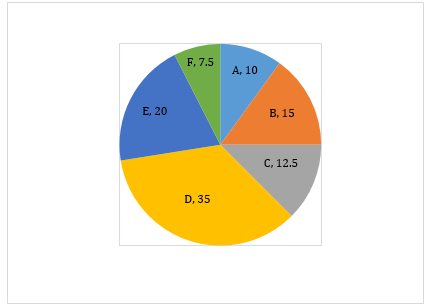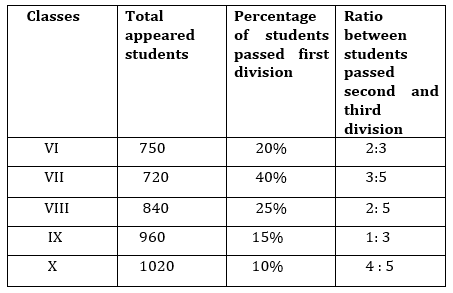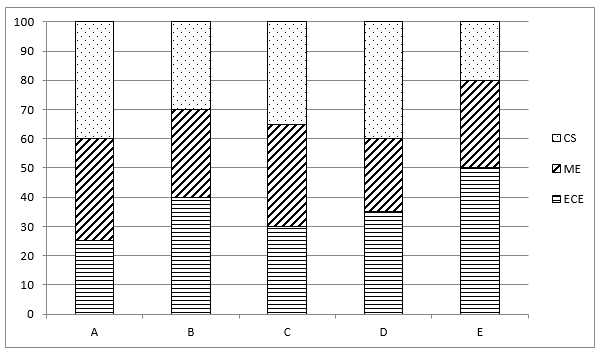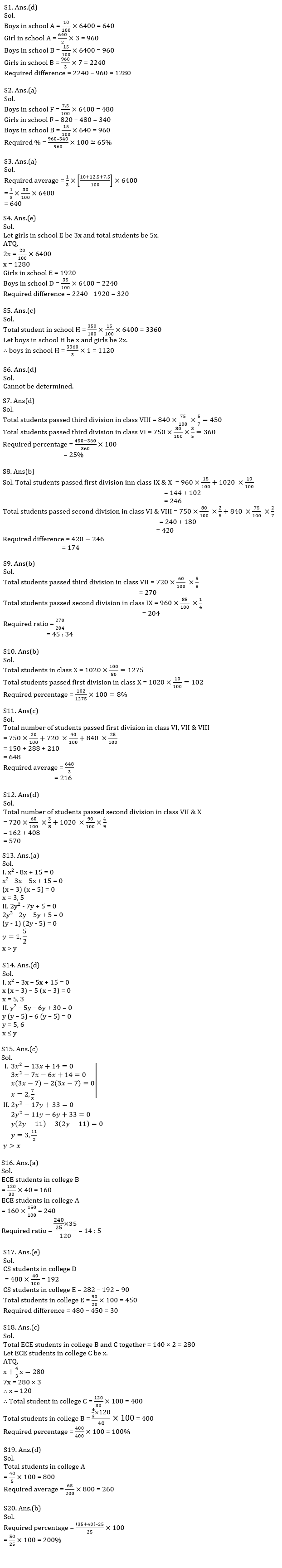Latest Banking jobs   »   Quantitative Aptitude Quiz For IBPS RRB...

# Quantitative Aptitude Quiz For IBPS RRB PO, Clerk Prelims 2021- 23rd May

Directions (1-6): Study the bar-graph given below & answer the questions.
Pie-chart given below shows the percentage distribution of total no. of boys in six different Schools.
Total Boys in all schools together = 6400Q1. If the ratio boys to girls in School A and School B is 2 : 3 and 3 : 7 respectively. Then find the difference of total girls in school B and A?
(a) 1020
(b) None of these
(c) 1160
(d) 1280
(e) 1240

Q2. If total no. of students in school F is 820. Then girls in school F is approximately what percent more/less than total boys in school B?
(a) 65%
(b) 74%
(c) 58%
(d) 78%
(e) 55%

Q3. What is the average of total no. of boys in school A, C and F?
(a) 640
(b) 680
(c) 720
(d) None of these
(e) 540

Q4. If ratio of girls to total students in school E is 3 : 5. Then find difference of boys in school D to girls in school E?
(a) 460
(b)420
(c) None of these
(d) 360
(e) 320

Q5. Total student in another school H is 250% more than the boys in school B and ratio of boys to girls in school H is 1 : 2. Then find number of boys in school H?
(a) 1140
(b) None of these
(c) 1120
(d) 1040
(e) 1210

Q6. If number of boys in school A and school D together is 2/5 th of the total students in both schools then find difference of girls in school A and school D?
(a) 820
(b) 680
(c) 520
(d) Cannot be determined
(e) None of these

Directions (7-12): Table given below shows total number of students appeared in five different class exams, percentage of students passed first division & ratio between students passed second and third division.Note – No student failed in exam and all student passed in three division only.

Q7. Total students passed third division in class VIII is what percent more than that of total students passed third division in class VI?
(a) 20%
(b) 15%
(c) 10%
(d) 25%
(e) 5%

Q8. Total students passed first division in class IX & X together is how much less than total students passed second division in class VI & VIII together?
(a) 166
(b) 174
(c) 154
(d) 172
(e) 184

Q9. Find the ratio between total students passed third division in class VII to total students passed second division in class IX?
(a) 45 : 38
(b) 45 : 34
(c) 45 : 44
(d) 45 : 32
(e) 45 : 28

Q10. If total students appeared in class X exam is 80% of total students in that class, then find total students passed first division in class X is what percent of total students in that class?
(a) 4%
(b) 8%
(c) 6%
(d) 10%
(e) 12%

Q11. Find the average number of students who passed first division in class VI, VII & VIII?
(a) 214
(b) 218
(c) 216
(d) 210
(e) 220

Q12. Find total number of students who passed second division in class VII & X together?
(a) 676
(b) 670
(c) 672
(d) 570
(e) 684

Directions (13-15): In each of these questions, two equations (I) and (II) are given. You have to solve both the equations and give answer

Q13.
I. x² – 8x + 15 = 0
II. 2y² – 7y + 5 = 0
(a) if x > y
(b) if x ≥ y
(c) if x < y
(d) if x ≤ y
(e) if x = y or no relation can be established between x and y.

Q14.
I. x² – 8x + 15 = 0
II. y² – 11y + 30 = 0
(a) if x > y
(b) if x ≥ y
(c) if x < y
(d) if x ≤ y
(e) if x = y or no relation can be established between x and y.

Q15.
I. 3 x²-13x+14=0
II. 2y²-17y+33=0
(a) if x > y
(b) if x ≥ y
(c) if x < y
(d) if x ≤ y
(e) if x = y or no relation can be established between x and y.

Directions (16-20): Study the following bar-graph carefully and answer the following questions.
Bar-graph given below shows the percentage distribution of number of engineering students according to their branch in five different colleges.Q16. If number of students in ME branch in college B is 120 and ECE students in college A is 50% more than ECE students in college B. Then find ratio of ME branch students in college A to that in college B ?
(a) 14 : 5
(b) 12 : 5
(c) 2 : 1
(d) 5 : 3
(e) 12 : 7

Q17. If total number of students in college D is 480 and CS branch students in college D and E together is 282. Then find difference between total students in college D and E ?
(a) None of these
(b) 20
(c) 50
(d) 25
(e) 30

Q18. If average number of ECE branch students in college B and C is 140 and ECE branch students in college B is 33 ⅓% more than ECE branch students in college C. Then total students in college B is what percent of total student in college C ?
(a) 50%
(b) 150%
(c) 100%
(d) 200%
(e) None of these

Q19. If difference between ME and CS student in college A is 40 then find average of ECE and CS students in this college?
(a) 240
(b) None of these
(c) 320
(d) 260
(e) 130

Q20. Number of ECE and CS branch students together in college D is what percent more/less than number of ME branch students in that college ?
(a) 300%
(b) 200%
(c) 100%
(d) None of these
(e) 150%

Practice More Questions of Quantitative Aptitude for Competitive Exams:

###### Study Plan for IBPS RRB PO/Clerk Prelims 2021

Solutions#### Congratulations!Incorrect details? Fill the form again here

•IBPS RRB Previous Year Question Paper PD...
•IBPS RRB Clerk 2nd Reserve List 2022 Out...
•Quantitative Aptitude Quiz For IBPS RRB ...
•Quantitative Aptitude Quiz For IBPS Cler...
•Reasoning Ability Quiz For IBPS RRB PO P...
•Quantitative Aptitude Quiz For IBPS RRB ...